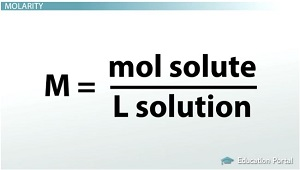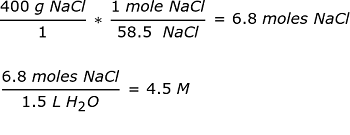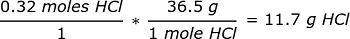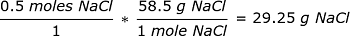# Calculating Molarity and Molality Concentration

An error occurred trying to load this video.

Try refreshing the page, or contact customer support.

Coming up next: Calculating Dilution of Solutions

### You're on a roll. Keep up the good work!

Replay
Your next lesson will play in 10 seconds
• 0:05 Concentration
• 0:49 Molarity
• 4:01 Molality
• 5:28 Lesson Summary
Save Save

Want to watch this again later?

Timeline
Autoplay
Autoplay
Speed Speed

#### Recommended Lessons and Courses for You

Lesson Transcript
Instructor: Amy Meyers

Amy holds a Master of Science. She has taught science at the high school and college levels.

Learn the abbreviations and meaning of molarity and molality. Then apply what you have learned by going over some sample calculations with given concentrations.

## Concentration

You have learned about solutions and that they are homogeneous mixtures of solutes in solvents. Solutions can have different amounts of solutes in solvents, which is known as concentration. Concentration is the amount of a substance in a given quantity of a solution.

Molarity and molality are both ways to express concentration. Molarity is abbreviated as 'M' and is the moles of solute per liter of solution.
M = mol solute / L solution.
Molarity is usually used in stoichiometric calculations involving solutions.

Molality is abbreviated as 'm' and is moles of solute per kilogram of solvent.
m = mol solute / kg solvent.

## MolarityWhen chemists are working in the lab, they often find it easier to deal with moles of a solute rather than the mass. Moles lets them know how many particles of the solute they have, and when they are working with balanced equations, knowing the exact number is easier for them. Calculating the molarity is easy. If you know the moles of solute and the amount of solution, you just divide them.

For example, if you have 0.5 moles of NaCl in 1.5 L of H2 O, the molarity is 0.5 / 1.5 L. M = 0.33 M.

If you don't know the moles but you have the mass, you can still figure out the molarity by using the mole ratio.

Pretend you have 400 g of NaCl in 1.5 L of H2 O.
From the periodic table, you know that one mole of NaCl has a mass of 58.5 g.Easy, right?

Here's a second example:

You have 0.8 L of a 0.4 M HCl solution. How many moles of HCl does the solution contain?

Molarity = mol solute / L solution
0.4 M = mole HCl / 0.8 L
0.32 = moles of HCl

Since you know the moles of HCl, can you determine the grams of HCl in that solution? Sure you can. From the periodic table, you know that one mole of HCl has a molar mass of 36.5 g.If you worked in a lab and your supervisor asked you to prepare one liter of 0.5 molar solution of NaCl, how would you go about doing that? All you would have to do is figure out how many grams of NaCl you need to add.To unlock this lesson you must be a Study.com Member.

### Register to view this lesson

Are you a student or a teacher?

#### See for yourself why 30 million people use Study.com

##### Become a Study.com member and start learning now.
Back
What teachers are saying about Study.com

### Earning College Credit

Did you know… We have over 200 college courses that prepare you to earn credit by exam that is accepted by over 1,500 colleges and universities. You can test out of the first two years of college and save thousands off your degree. Anyone can earn credit-by-exam regardless of age or education level.